# Probability Toss

5th graders practise probability by using their knowledge of fractions with these probability toss worksheets.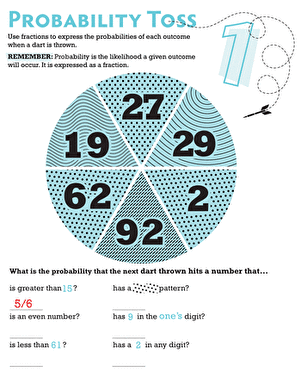### Probability Toss 1

What are the chances that a dart will land on an even number on a dart board? Find out in this sixth grade probability worksheet!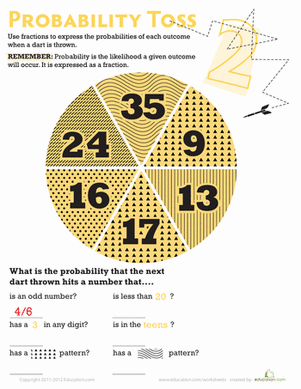### Probability Toss 2

Boost your child's maths know-how! What are the odds a dart would land on one of the given sections of this circle?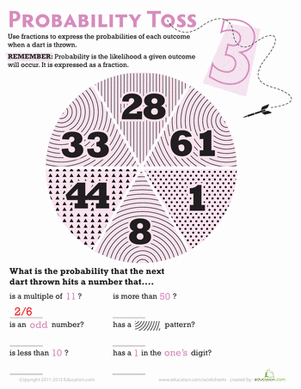### Probability Toss 3

If your sixth grader likes darts, then he'll love this probability worksheet! This dart board themed worksheet introduces your child to simple probability.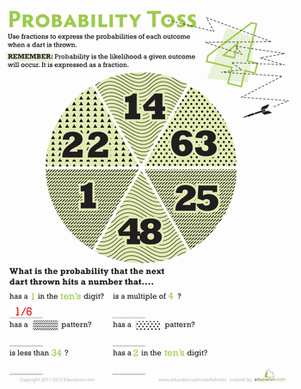### Probability Toss 4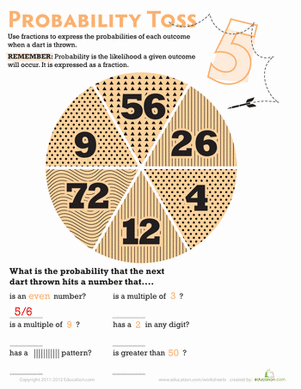### Probability Toss 5

Sixth graders will practise probability in this dart board themed worksheet. Your kid will calculate the probability of a dart hitting a certain kind of number.

Create new collection

0

### New Collection>

0Items

What could we do to improve Education.com?